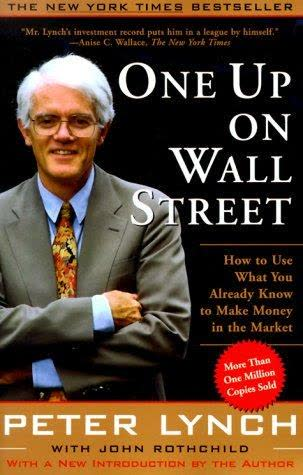Select Page

# PEG Ratio: Useful or Obsolete Valuation Metric?The PEG Ratio is a stock valuation tool for investment analysis. The purpose of the PEG Ratio is to evaluate and the trade off between the price of a stock, the earnings per share, and the company’s expected growth rate.

Peter Lynch, when managing the ultra successful Fidelity Magellan mutual fund in the 80’s & 90’s, popularized the ratio. He writes in his book “One Up On Wall Street”: “The P/E ratio of any company that’s fairly priced will equal its growth rate.”

## PEG Ratio Calculation

The PEG Ratio calculation is:

PE Ratio (Price/Earnings) / Expected Growth Rate = PEG Ratio

## How to Use the PEG Ratio

Lynch recommended that you first find attractive companies, and then use the PEG ratio as a tool to evaluate and compare the different companies.

In general, companies with high PEG ratios (over 1.0) would be more overvalued than companies with low PEG ratios (under 1.0).

Examples:

Company A is selling for \$30, has earnings of \$2, and an expected growth rate of 15%. PEG Ratio =
(\$30 / 2 ) = \$15 / 15 = 1

Company B is selling for \$60, has earnings of \$4, and an expected growth rate of 20%. PEG Ratio =
(\$60 / 4 ) = \$15 / 20 = 0.75

Company C is selling for \$45, has earnings of \$1, and an expected growth rate of 30%. PEG Ratio =
(45 / 1 ) = \$45 / 30 = 1.5

The PEG Ratio would indicate company B has the most undervalued price. Company C is the fastest grower but the most overvalued price. Company A is expected to grow the slowest and is fairly valued.

The main value of the PEG ratio is that it is an easy calculation and assigns a relative value to expected future earnings growth of a company. In other words, it allows the analyst to compare the valuation of companies with different growth rates. It is a more thorough picture of valuation than the P/E ratio alone.

The PEG ratio is rule of thumb, or an approximation; not a mathematical certainty by any means. In addition, the formula is only as good as its inputs.

The greatest chance for inaccurate input would be the future growth rate assumption. Anytime an analyst makes assumptions about the future it can be incorrect.

The PEG ratio has limitations in measuring companies with low growth. As an example, a mature company may have good earnings and a solid dividend, but a slow growth rate. Obviously, a company growing earnings at 1% is probably not going to sell at a P/E ratio of 1.

The PEG ratio is also highly susceptible to large errors for fast growing companies. For example, a company growing in excess of 25% per year will be unable to sustain such a growth rate. Therefore you have to make assumptions in growth rates that are speculative. Again, the PEG ratio is only as good as its inputs.

## Useful or Obsolete Valuation Metric

Several decades ago, when Peter Lynch popularized this ratio, simple metrics such as the PEG ratio served a valuable purpose; providing valuation guidelines for investors to follow in an era when metrics weren’t easily accessible. The trade off between price and growth is a concept every investor should understand.

However, with the ease of access to many different valuation metrics through online sources and services, the value of the PEG ratio has been diminished. The usefulness of this single metric no longer has the gravitas it once did.